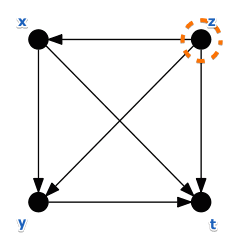# ML Wiki

## Voting Theory Examples

Different examples from Voting Theory that illustrate some interesting properties.

## Example 1

This is an example in which different voting mechanisms of Voting Theory produce different results.

Consider this example:

• $N = 24$
• $A = \{ t, x, y, z \}$

• 5 votes $x > y > z > t$
• 4 votes $x > z > y > t$
• 2 votes $t > y > x > z$
• 6 votes $t > y > z > x$
• 8 votes $z > y > x > t$
• 2 votes $t > z > y > x$

### Example 1: Plurality Voting

The global ranking is

$t > x > z > y$
$(10 > 9 > 8 > 0)$

Therefore $t$ gets elected

• But 17 voters (the majority!) prefer $x$ to $t$
• Condorcet Fairness criterion is not satisfied

### Example 1: Two-Round Voting

Round 1:

• $x$ and $t$ are selected

Round 2:

• remove all other candidates:
• 5 + 4 + 8 = 17 votes $x > t$
• 2 + 6 + 2 = 10 votes $t > x$

$x$ wins

• but $y$ is preferred to $x$ by 18 voters (the majority!)
• Condorcet Fairness criterion is not satisfied

### Example 1: Borda's Rule

The Borda Scores:

• $B(t) = 57$
• $B(x) = 5 \cdot 4 + 4 \cdot 4 + 2 \cdot 2 + 6 \cdot 1 + 8 \cdot 2 + 2 \cdot 1 = 64$
• $B(y) = 75$
• $B(z) = 74$

$y$ wins

### Example 1: Condorcet's Rule

Pairwise comparison:

• $t,x: n_{xt} = 17, n_{tx} = 10 \Rightarrow x > t$
• $x,y: n_{xy} = 9, n_{yx} = 18 \Rightarrow y > x$
• $t,y: n_{ty} = 10, n_{yt} = 17 \Rightarrow y > t$
• $x,z: n_{xz} = 11, n_{zx} = 16 \Rightarrow z > x$
• $y,z: n_{yz} = 13, n_{zy} = 14 \Rightarrow z > y$
• $t,z: n_{tz} = 10, n_{zt} = 17 \Rightarrow z > t$

We build the preference graph for this:We see that $z$ is the winner